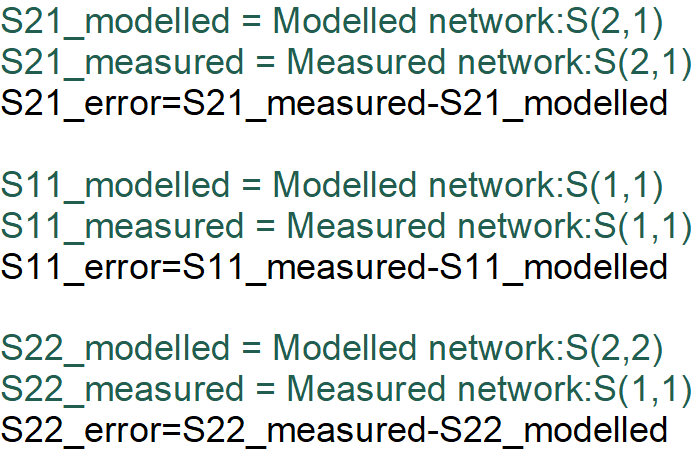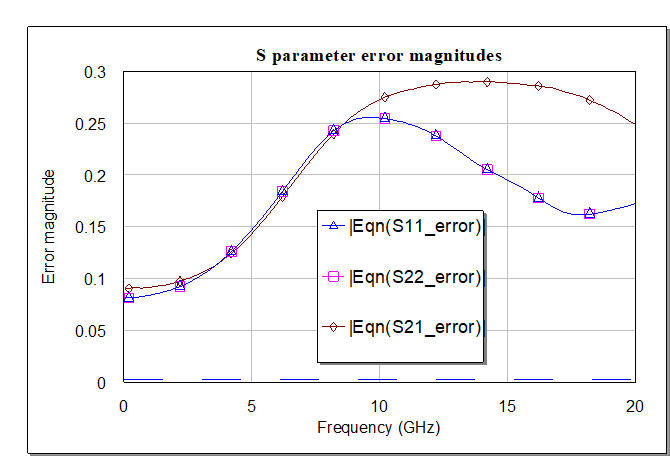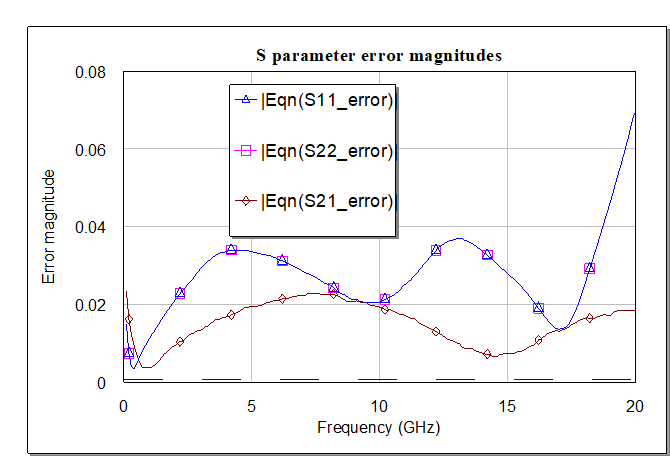# Equivalent circuit model fitter

New for June 2022. Let's talk about modeling! Fitting equivalent circuit models to measured S-parameters is a key skill for microwave design. Most device models inside simulations are based on fitted models, and some highly-paid individual had to do that dirty work. Well, in the case of high-end foundries, it may be done by artificial intelligence at this point, every job has its half-life. But in the real world, you will need to be able to fit models yourself, to whatever arbitrary structure is standing between you and success. Here you will learn how to fit a two-port model to measured data.

We have prepared a MWO file that will help you fit a two-port circuit to two-port data.  The data might not be measured at all.... it could be data from a 3D EM simulation.

Should you optimize to magnitudes of S-parameters, and angles separately? Should you optimize to scalar magnitudes or magnitudes in dB? The answers to both questions is "no". You need to optimize to complex S-parameters to get the best results. You may end up weighting various S-parameters differently, but that is part of the ol' black magic of modeling.

In the modeling file, you will have two blocks, one that is the "modelled network" and one that is the "measured network".  Here are  equations that subtract complex S-parameters of the model from the measurement. We assumed your data is passive so we ignored S12 as it should be the same as S21 (it is easy to add  yourself if you need it).Note that we plot and optimize the magnitude of the error vectors, which means the maximum error for a passive network is a value of 2 (from one side of the Smith chart to the opposite). We optimize the errors to a goal of zero. If the errors show a negative value, you don't know the difference between "real" and "magnitude".

Below is an example plot of error magnitudes, at the start of the model fit. The error magnitudes are off by almost 0.3 at some frequencies, which is a sizable error.Here the optimizer has been run until stagnation. The errors are quite small. We can all agree that this is a good fit.Now click here to see an example of the model fitter in action, fitting a model to a broadband gain equalizer.

Author : Unknown Editor